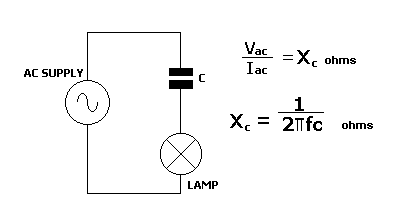Home > Electronic Tutorials > Resistance and Impedance in AC Circuit > Capacitive in an AC Circuit Tutorial

Resistance and Impedance in AC Circuit

# Capacitive in an AC Circuit TutorialThe capacitor opposes the flow of ac current, as a resistor does in a dc circuit.

This opposition is called capacitive reactance, Xc. It is measured in ohms. Ohm's Law can be applied, as in the top formula.

The bottom formula shows how capacitive reactance is calculated.

f is the frequency of the applied voltage, and C is the value of the capacitor in Farads.

It can be seen from this formula that the value of Xc goes down as the frequency increases. It also goes down if the value of the capacitor increases.

This that as the value of C or f increases, the opposition to the flow of ac current decreases, and the lamp will glow brighter.